Courses

# RD Sharma Solutions (Part - 1) - Ex-20.4, Mensuration - I, Class 7, Math Class 7 Notes | EduRev

## Class 7: RD Sharma Solutions (Part - 1) - Ex-20.4, Mensuration - I, Class 7, Math Class 7 Notes | EduRev

The document RD Sharma Solutions (Part - 1) - Ex-20.4, Mensuration - I, Class 7, Math Class 7 Notes | EduRev is a part of the Class 7 Course RD Sharma Solutions for Class 7 Mathematics.
All you need of Class 7 at this link: Class 7

#### Question 1:

Find the area in square centimetres of a triangle whose base and altitude are as under:
(i) base = 18 cm, altitude = 3.5 cm
(ii) base = 8 dm, altitude = 15 cm

We know that the area of a triangle = 1/2×Base×Height
(i) Here, base = 18 cm and height = 3.5 cm
∴ Area of the triangle = (1/2×18×3.5)=31.5 cm2
(ii) Here, base = 8 dm = (8 x 10) cm = 80 cm     [Since 1 dm = 10 cm]
and height = 3.5 cm
∴ Area of the triangle = (1/2×80×15)=600 cm2

#### Question 2:

Find the altitude of a triangle whose area is 42 cm2 and base is 12 cm.

We have,
Altitude of a triangle =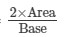Here, base = 12 cm and area = 42 cm2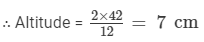#### Question 3:

The area of a triangle is 50 cm2. If the altitude is 8 cm, what is its base?

We have,
Base of a triangle =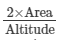Here, altitude = 8 cm and area = 50 cm2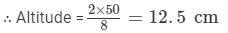#### Question 4:

Find the area of a right angled triangle whose sides containing the right angle are of lengths 20.8 m and 14.7 m.

In a right-angled triangle, the sides containing the right angles are of lengths 20.8 m and 14.7 m.
Let the base be 20.8 m and the height be 14.7 m.
Then,
Area of a triangle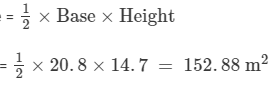#### Question 5:

The area of a triangle, whose base and the corresponding altitude are 15 cm and 7 cm, is equal to area of a right triangle whose one of the sides containing the right angle is 10.5 cm. Find the other side of this triangle.

For the first triangle, we have,
Base = 15 cm and altitude = 7 cm
Thus, area of a triangle =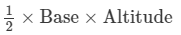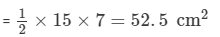It is given that the area of the first triangle and the second triangle are equal.
Area of the second triangle = 52.5 cm2
One side of the second triangle = 10.5 cm
Therefore,
The other side of the second triangle =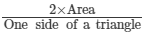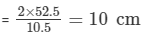Hence, the other side of the second triangle will be 10 cm.

#### Question 6:

A rectangular field is 48 m long and 20 m wide. How many right triangular flower beds, whose sides containing the right angle measure 12 m and 5 m can be laid in this field?

We have,
Length of the rectangular field = 48 m
Breadth of the rectangular field = 20 m
Area of the rectangular field = Length x Breadth = 48 m x 20 m = 960 m2

Area of one right triangular flower bed = 1/2×12 m×5 m = 30 m2
Therefore,

Required number of right triangular flower beds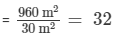#### Question 7:

In Fig. 29, ABCD is a quadrilateral in which diagonal AC = 84 cm; DL ⊥ ACBM ⊥ ACDL = 16.5 cm and BM= 12 cm. Find the area of quadrilateral ABCD.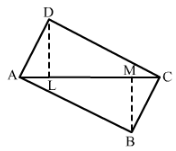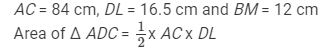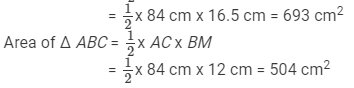Hence,
Area of quadrilateral ABCD = Area of Δ ADC  + Area of Δ ABC
= (693 + 504) cm2
= 1197 cm

#### Question 8:

Find the area of the quadrilateral ABCD given in Fig. 30. The diagonals AC and BD measure 48 m and 32 m respectively and are perpendicular to each other.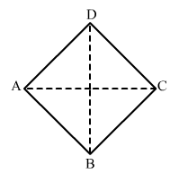We have,
Diagonal AC = 48 cm and diagonal BD = 32 m
∴ Area of a quadrilateral = 1/2 x Product of diagonals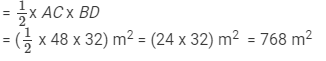#### Question 9:

In Fig 31, ABCD is a rectangle with dimensions 32 m by 18 m. ADE is a triangle such that EF ⊥ AD and EF= 14 cm. Calculate the area of the shaded region.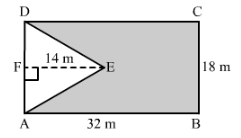We have,
Area of the rectangle = AB x BC
= 32 m x 18 m
= 576 m2

Area of the triangle  =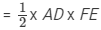= 1/2x BC x FE       [Since AD = BC]
= 1/2x 18 m x 14 m
= 9 m x 14 m = 126 m2
∴ Area of the shaded region = Area of the rectangle − Area of the triangle
=(576 − 126) m2
= 450

#### Question 10:

In Fig. 32, ABCD is a rectangle of length AB = 40 cm and breadth BC = 25 cm. If PQRS be the mid-points of the sides ABBCCD and DA respectively, find the area of the shaded region.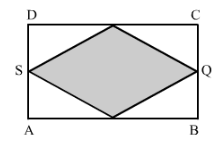We have,
Join points PR and SQ.
These two lines bisect each other at point O.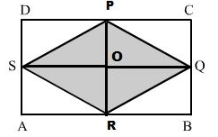Here, ABDCSQ = 40 cm and AD = BC =RP = 25 cm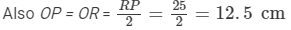From the figure we observed that,
Area of Δ SPQ = Area of Δ SRQ
Hence, area of the shaded region  2 x (Area of Δ SPQ)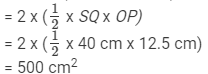The document RD Sharma Solutions (Part - 1) - Ex-20.4, Mensuration - I, Class 7, Math Class 7 Notes | EduRev is a part of the Class 7 Course RD Sharma Solutions for Class 7 Mathematics.
All you need of Class 7 at this link: Class 7Use Code STAYHOME200 and get INR 200 additional OFF Use Coupon Code
All Tests, Videos & Notes of Class 7: Class 7

### Up next### Top Courses for Class 7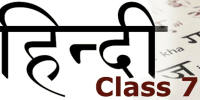## RD Sharma Solutions for Class 7 Mathematics

97 docs

### Up next### Top Courses for Class 7Track your progress, build streaks, highlight & save important lessons and more!

,

,

,

,

,

,

,

,

,

,

,

,

,

,

,

,

,

,

,

,

,

,

,

,

,

,

,

,

,

,

;